# Hybrid (3 dB) couplers

Click here to go to our main page on couplers and power splitters

Click here to learn why there is no way to make a 45 degree hybrid coupler, thanks to Nick! (New for March 2020)

Hybrid couplers are the special case of a four-port directional coupler that is designed for a 3-dB (equal) power split. Hybrids come in two types, 90 degree or quadrature hybrids, and 180 degree hybrids. Why isn't there a "45 degree hybrid" you ask? Maybe it wouldn't isolate the fourth port!  New for March 2020: here's proof there is no way to make a 45 degree hybrid coupler, thanks to Nick!

Hybrid couplers are often used in creating reflection phase shifters.

All of the couplers discussed on this page have separate pages that go into detail on their operation. This page helps tie the entire mess together.

### 180 degree hybrid couplers

These include rat-race couplers and waveguide magic tees. Here we will look at the rat-race and introduce the vector and shorthand notation that is often used when referring to 180 degree hybrid couplers. In the figures, the skinny lines have impedance 1.41xZ0.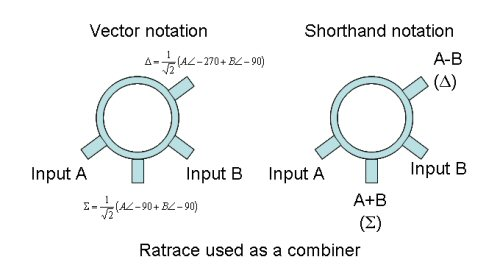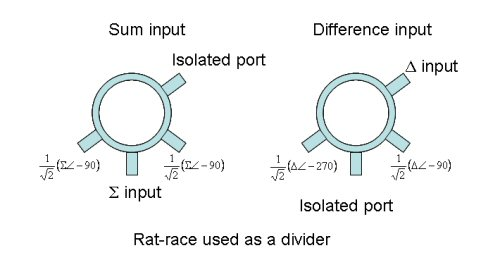Let's look at the S-parameters of a "classic" rat-race response (equal split at center frequency). Oops, we forgot to number the ports in the figure above, and Dave just reminded us (May 2013). So we created a MWO schematic to define the port numbers, and then plotted the S-parameters.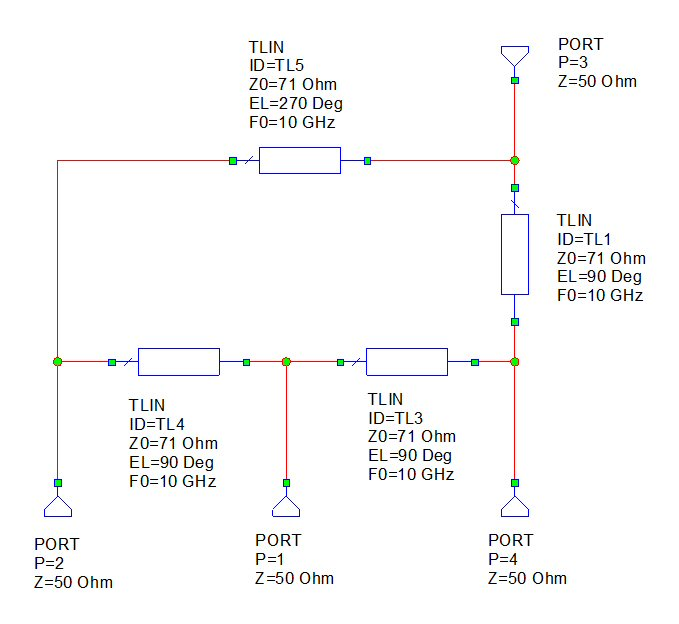There are no conventions on how to number the ports, we started numbering them clockwise from the Sum port (port 1). The 3 dB ports are 2 and 4 when driven by either port 1 or port 3, (which are isolated from each other). By symmetry (look at the sketched layouts, not the schematic), you could also say that ports 1 and port 3 are 3 dB ports, when driven by either port 2 or port 4 (which are also isolated from each other).

Below is the response, when you are exciting port 3 (the delta port). We included the phase difference between ports 2 and 4 to show that it is -180 degrees at band center.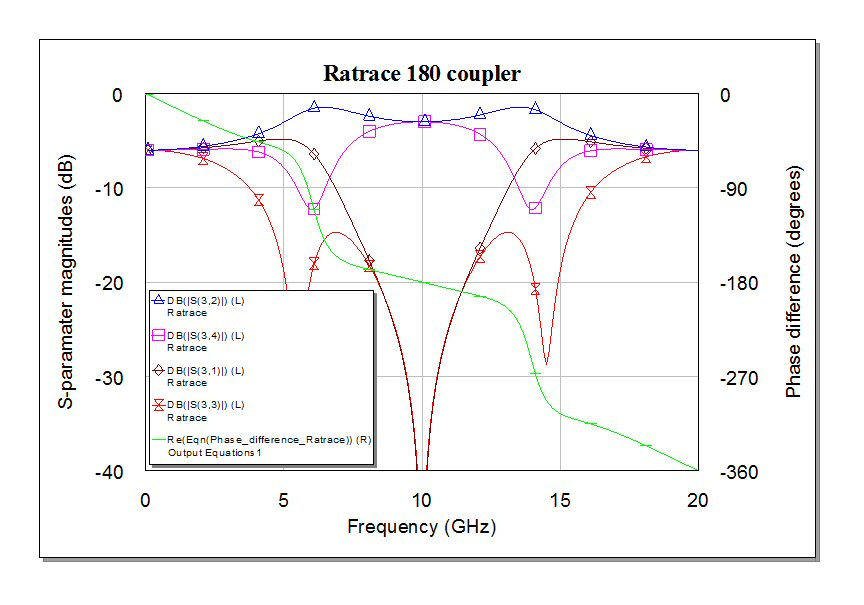Here is the MWO equation block in case you were wondering how we calculated the phase difference between ports 2 and 4 when driven by port 3. The blue letters are "measurements" while the black letters are calculations. Note that when you "measure" the phase angles in this manner MWO reports them in radians, so we converted the angle difference to degrees in the equation.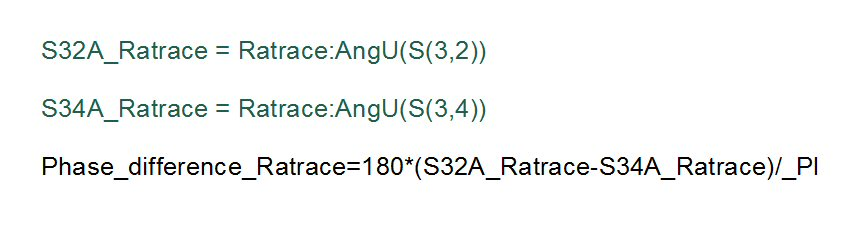The rat-race gives about 32% bandwidth for a phase error of +/-10 degrees from the ideal 180 degree split.

### 90 degree hybrid couplers

These are often called quadrature couplers, and include Lange couplers, the branchline coupler, overlay couplers, edge couplers, and short-slot hybrid couplers. Here we will just look at a branchline, and show you some of the "short hand" notation that is often used when referring to 90 degree hybrids.

Below the branchline is used as a combiner. The input signals are vectors of magnitude A and B, then the outputs are as shown. Note that because we are dealing with voltages, the outputs have a square-root-of-two factor. Power into either "input" on the left is split exactly in half on the "outputs" on the right (-3 dB), equal to the square of the voltage split. In the figure, the fat lines have impedance of 0.707xZ0.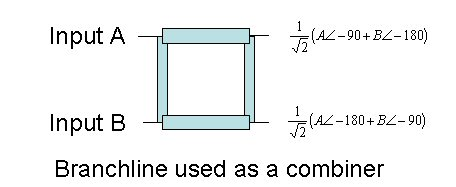Now let's look at it as a divider. Here only an input signal is present at port A. It splits by 3 dB at the two outputs, and is isolated from Port B (ideally zero energy comes out this port).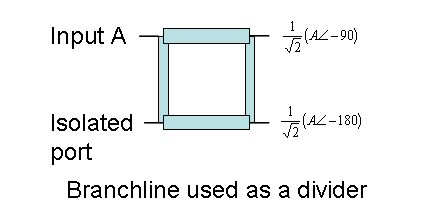### Converting a branchline coupler to a 180 degree hybrid

In some applications like a monopulse comparator, available 180 degree hybrids complicate the layout because the "sum" port is between the split ports. But it is as easy as adding a 90 degree section to one of the ports of a branchline. Below we've lengthened "Input A" by a quarter-wavelength (impedance is Z0). Now we've got a sum and a difference output, just like a rat-race.

This image was corrected on March 28, 2008, previously the short-hand notation was wrong (our apologies for the error). Thanks to Lyn and Bernhard who both pointed this out!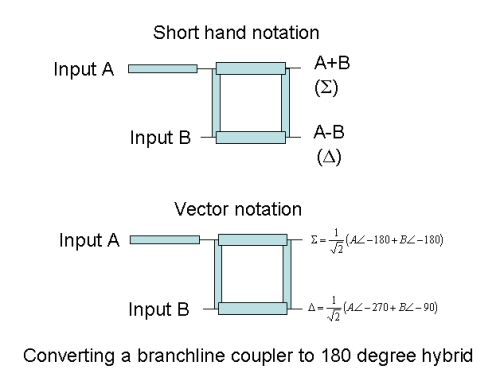Now let's look at the response of this component, and compare it to the "classic" rat-race. We show a Microwave Office schematic below, where we started numbering with Port 1 on the odd-looking leg and went clockwise. The Delta port is (again) port 3, so maybe there is a method to this madness. Note that if you drove the splitter from either port on the left (port 1 or port 4) it reverts to a 90 degree hybrid!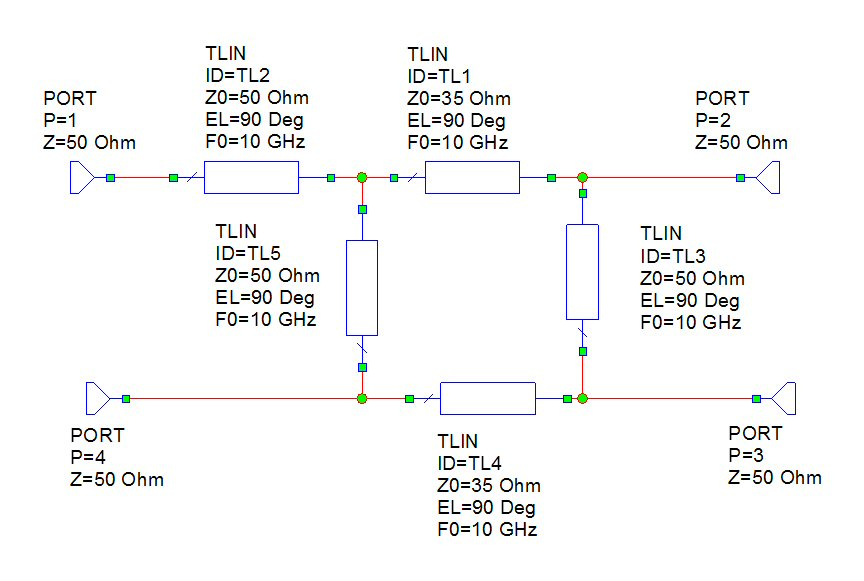The 3 dB split ports in the 180 case are port 1 and port 4 and the isolated ports are port 2 and port 3. Note that port 1 and port 4 are also isolated from each other.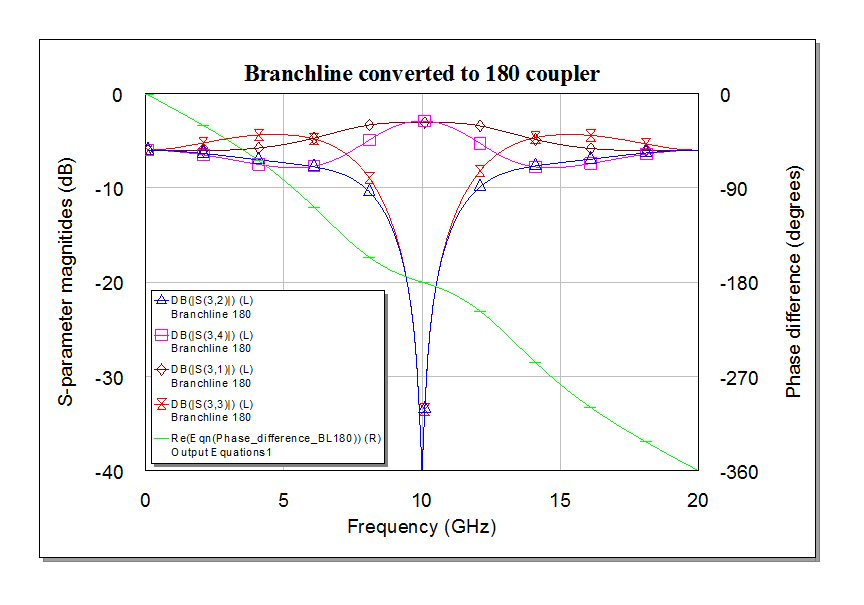And again, here is the MWO equation block if you are interested.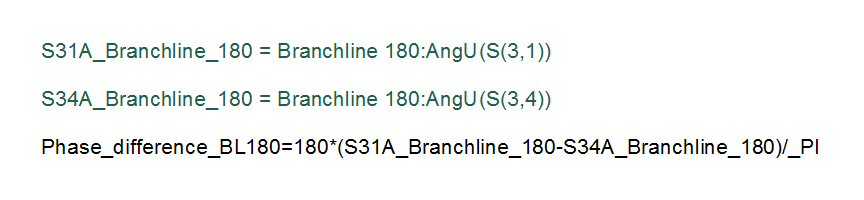The bandwidth is less. If we just look at the frequency where the 180 degree split is within +/- 10 degrees, it is about 20% (0.9 to 1.1 GHz). Referring to the classic rat-race above, it has 32 percent bandwidth for the same phase error. The 180 degree branchline is a poor substitute for a rat-race, when you look at other parameters such as impedance match and isolation bandwidth.

### Using back-to-back hybrids to achieve an RF-RF crossover

By cascading two hybrid couplers, you can create a four-port network that provides isolation between two RF paths that cross each other (as opposed to an airbridge or wirebond crossover). This should only be attempted where there is plenty of room for it, and it is probably useful over only 10% bandwidth.

Here's a schematic: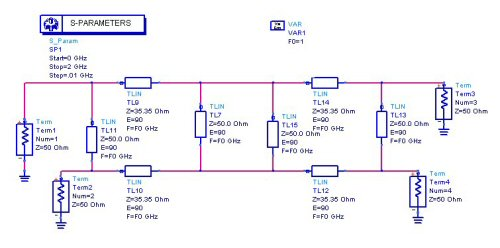And here's the response. S41 is the path that is coupled, S21 and S31 are isolated, at least at the center frequency (in this case 1 GHz). This structure has a severe bandwidth limitation. In most cases you should consider a 3-dimensional RF crossover first, like an airbridge or a wirebond, before you resort to this!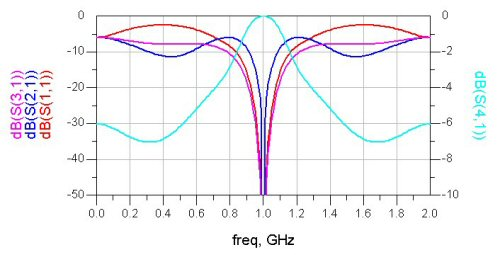Author : Unknown Editor